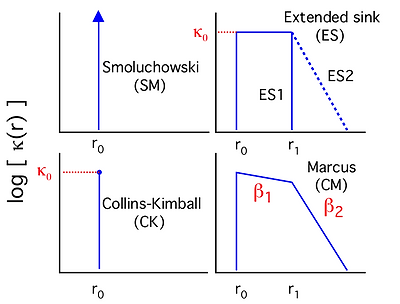# Diffusion-Reaction Models in Ionic Liquids

In order to obtain a more sophisticated description to photo-induced electron transfer reactions, diffusion-reaction (DRE) models are used to interpret the fluorescence quenching of a photo-excited electron acceptor by electron donors.

DRE models are solutions to symmetric spherical diffusion-reaction equation under different boundary conditions. We used four different models in this work, they correspond to different forms of a distance dependent electron transfer rate κ(r). ( κ(r) is the exact electron transfer rate when donor and acceptor is at a distance r, which is different from the fluorescence quenching rate kq. kq contains the contribution of diffusion.) In the Smoluchowski and Collins-Kimball modes, the electron transfer is assumed to occur only at a single distance r0. The extended sink model assumes κ(r) is nonzero over a finite thickness first solvation shell, and decreases exponentially beyond the first shell. The Marcus model describes κ(r) using classical Marcus theory. The later two modes are based on an approximate solution to the diffusion-reaction equation by Dudko and Szabo.The models are applied on time-dependent fluorescence decay K(t) measured by experiments. K(t) is defined by the log of time resolved fluorescence decay, divided by the concentration of electron donor. We also defined a parameter ρ to indicate "goodness" of the fits, where ρ=1 indicates a perfect fit, and a large ρ value indicates a poor fit. In acetontrile, all modes can describe K(t) well with a small ρ value. In contrast, only extend sink and classical Marcus models fit experimental K(t) in ionic liquids. Therefore, we conclude that a distance-dependent electron transfer rate is required for modeling the reactions in ionic liquids.In our classical Marcus model, where κ(r) is computed using Marcus theory, by fitting the electron transfer quenching data, we are able to extract the diffusion coefficients of the reactants and the reaction free energy. The following graph shows comparison of diffusion coefficients D and free energy ΔG0 obtained from DRE fits and estimated from PG-SE NMR or electrochemistry measurements. The blue dots show the reactions where neutral donors are used, and for red dots the donors are anions. There is a good match between D and ΔG0 obtained from fits and experiments.